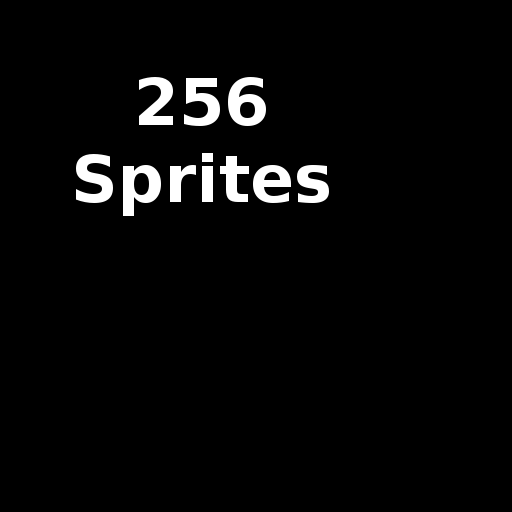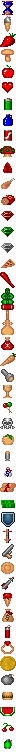# sprites256

This short example shows the maximum number of hardware sprites moving on screen.

First it loads a background image into character RAM:then loads 47 sprite images into sprite image RAM:The loop moves all 256 sprites, bouncing then off the screen edges.

```#include <SPI.h>
#include <GD.h>

#include "pickups2.h"
#include "sprites256.h"

struct sprite
{
int x;
int y;
char vx, vy;
} sprites;

void plot()
{
int i;
for (i = 0; i < 256; i++) {
GD.sprite(i, sprites[i].x >> 4, sprites[i].y >> 4, i % 47, 0, 0);
}
}

#define LWALL (0 << 4)
#define RWALL (384 << 4)
#define TWALL (0 << 4)
#define BWALL (284 << 4)

void move()
{
int i;
sprite *ps;

for (i = 256, ps = sprites; i--; ps++) {
if ((ps->x <= LWALL)) {
ps->x = LWALL;
ps->vx = -ps->vx;
}
if ((ps->x >= RWALL)) {
ps->x = RWALL;
ps->vx = -ps->vx;
}
if ((ps->y <= TWALL)) {
ps->y = TWALL;
ps->vy = -ps->vy;
}
if ((ps->y >= BWALL)) {
ps->y = BWALL;
ps->vy = -ps->vy;
}
ps->x += ps->vx;
ps->y += ps->vy;
}
}

void setup()
{
int i;

GD.begin();

GD.copy(RAM_PIC, sprites256_pic, sizeof(sprites256_pic));
GD.copy(RAM_CHR, sprites256_chr, sizeof(sprites256_chr));
GD.copy(RAM_PAL, sprites256_pal, sizeof(sprites256_pal));

GD.copy(RAM_SPRIMG, pickups2_img, sizeof(pickups2_img));
GD.copy(RAM_SPRPAL, pickups2_pal, sizeof(pickups2_pal));

for (i = 0; i < 256; i++) {
sprites[i].x = random(400 << 4);
sprites[i].y = random(300 << 4);
sprites[i].vx = random(-16,16);
sprites[i].vy = random(-16,16);
}
}

void loop()
{
plot();
move();
}
```

Thanks to SpriteLib GPL for the sprite images.